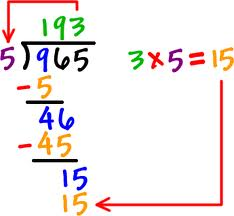Strategy. Innovation. Brand.

# Meaningless MeansQuick. What’s the mean?

The distance between 78 and 79 is the same as the distance between 96 and 97.

It may seem like a simple answer but it’s actually rather subtle. As with all good questions, the answer is “It depends”.

Let’s say that you’re measuring a distance in meters. You’re standing at meter zero. On a straight line, Object A is 78 meters away from you, Object B is 79 meters away, Object C is 96, and Object D is 97. Is the distance between Object A and B the same as the distance between Objects C and D?

Well, yes it is. After all a meter is a meter. A meter has a standard, universal definition. In fact, it’s one ten-millionth of the distance from the North Pole to the equator, on an arc passing through Paris*. (Those pesky French defined it). Because we have a standard and objective way of measuring a meter, we can safely conclude that the distance between A and B is the same as that between C and D.

Let’s look at a different example: ice dancing in the Olympics. The winners receive Gold, Silver, and Bronze medals. Is the distance between Bronze and Silver the same as the distance between Silver and Gold? Well, no. For one thing, we don’t have a standard, objective way to measure. Judging ice dancing is subjective. The Russian and American judges may well have different points of view.

Additionally, most athletes would say that improving performance becomes increasingly difficult the closer you get to the top. Let’s say that you can run a mile in ten minutes. You work hard, improve your speed, and get your time down to nine minutes. (Congratulations). Let’s compare your level of effort to Mary who runs a six-minute mile and improves her time to five minutes. You’ve shaved a minute off your time and she’s shaved a minute off her time but, really, who has to work harder? I think we’d all agree that it’s Mary. So, the distance between ten minutes and nine minutes is not the same as that between six minutes and five.

Now, let’s calculate some means. Let’s say we have three buildings. One is 30 meters tall, the second is 60, and the third is 70. What’s the mean? We add up the numbers (160) and divide by the number of numbers (3) and find that the mean is 53.3 meters. Does that make sense? Sure it does, because we have a standard, objective way to measure a meter and the points on the scale are equidistant.

Let’s do the same for ice dancing medals. We’ll give three points to a gold medal, two to a silver, and one to a bronze. Now we can add up the numbers, divide by the number of numbers, and calculate a mean. Does it make sense? Well, no. There’s a little sleight of hand here. We’ve changed from rankings that are not equidistant (gold, silver, bronze) to numbers that are equidistant. It’s a bit slippery. It’s the kind of calculation that you might expect from major banks rather than from good researchers.

What does this have to do with your grade point average? Well, your GPA is much more like ice dancing medals rather than meters. The distance between and F and a D is much shorter than the distance between a B and an A. Since the points on the scale are not equidistant, it doesn’t make sense to calculate a mean. Remember that the next time someone asks your GPA.

*Actually, the definition of a meter has changed since it originated. Here’s a good explanation.